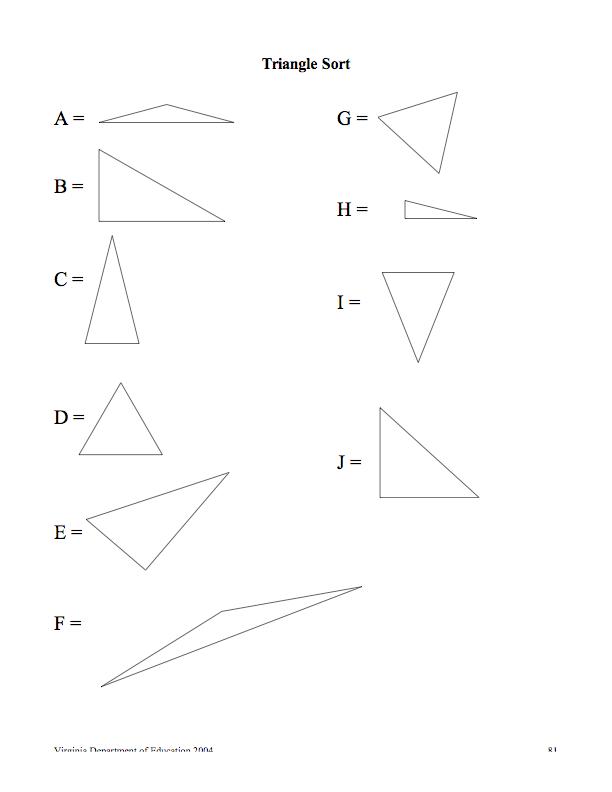# Reporting Category Geometry Topic Classifying and sorting angles and triangles Primary sol

Download 55.82 Kb.
 Date conversion 27.04.2018 Size 55.82 Kb.

Mathematics Enhanced Scope and Sequence – Grade 5

Triangle Sort

Reporting Category Geometry

Topic Classifying and sorting angles and triangles

Primary SOL 5.12 The student will classify

a) angles as right , acute, obtuse, and straight; and

b) triangles as right, acute, obtuse, equilateral, scalene, or isosceles.

Related SOL 5.11

## Materials

• A variety of pre-cut triangles with different angles and sides

• Triangle Sort activity sheet (attached)

• Triangle Sort recording sheet (attached)

• Protractors

## Vocabulary

angle, triangle, polygon, right, acute, obtuse, equilateral, scalene, isosceles

## Student/Teacher Actions (what students and teachers should be doing to facilitate learning)

1. Begin with a review of angles, asking students what they know about them and what types of angles they know. Review right, acute, obtuse, and straight angles.

1. Ask students for a definition of a triangle. Lead a discussion on the characteristics of a triangle (a polygon with three sides and three angles). Display three different triangles (e.g., one equilateral, one isosceles, one scalene). Have students compare and contrast the characteristics of triangles.

2. Explain that triangles can be classified according to the measures of their angles and the lengths of their sides. Ask the class to describe how triangles might differ in angle measures (right, acute, obtuse) and in length of their sides (scalene—no sides congruent; isosceles—at least two sides congruent; equilateral—all sides congruent).

3. Present students with the Triangle Sort activity sheet (attached), and explain that they will be sorting the triangles based on the measure of their angles and the length of the sides.

4. Begin with triangle A. Ask students to describe what they know about triangle A. Ask, “What types of angles do you see in triangle A? What can you say about the sides?” Allow students to measure sides and angles. Instruct students to write A under obtuse and under isosceles on the Triangle Sort Recording Sheet (attached). Demonstrate how to measure the sides. Do several more examples until your students are familiar with the process.

5. Pair students, and have them discuss each triangle and decide the appropriate places for it on the recording sheet. Encourage students to use the angle ruler and/or a ruler and protractor to measure the angles and sides.

6. When students finish with their partners, come back as a whole group and check and compare results.

7. Have students draw conclusions about the triangles. Ask students, “How are all the right triangles similar? How would you describe a scalene triangle? Can you have an isosceles right triangle? Can you have an isosceles obtuse triangle? Can you have an obtuse right triangle?”

8. Have students write their own definitions for each type of triangle.

## Assessment

• Questions

• What are some ways to sort or classify triangles? Give examples for each type of triangle you describe.

• Triangles can be named by the lengths of their sides and by the measures of their angles. If a triangle is acute, can it also be scalene? If a triangle is right, can it also be equilateral? What types can a triangle be simultaneously? Which types can a triangle not be at the same time?

• Journal/Writing Prompts

• Identify places we find triangles in nature and the built environment, and tell what kinds of triangles they are. List as many ideas as you can.

• Describe the six types of triangles. Explain how they are different.

• Other

• Ask students to draw a triangle with two right angles. Have them explain their results.

• Ask students to draw an equilateral triangle with an obtuse angle. Have them explain their results.

## Extensions and Connections (for all students)

• Have students go on a triangle hunt. Have students use digital cameras to take pictures of triangles they find inside and outside the school. Download them to a computer and embed them into an electronic presentation. Have students identify the types of triangles they found.

• Have students use geoboards to make and classify angles and triangles and to describe and analyze their properties.

• Have students bend straws to make each type of triangle. Tape them to poster board, and label them based on sides and angles.

## Strategies for Differentiation

• When introducing types of triangles, focus on one parameter at a time. Have students identify triangles based on only angles first, then only on sides. Once students are comfortable with each, lead students to categorize triangles by both sides and angles.

## Triangle Sort## Triangle Sort Recording Sheet

Name Date

 Acute Obtuse Right Scalene Equilateral Isosceles

Virginia Department of Education © 2011

The database is protected by copyright ©dentisty.org 2016
send message

Main page